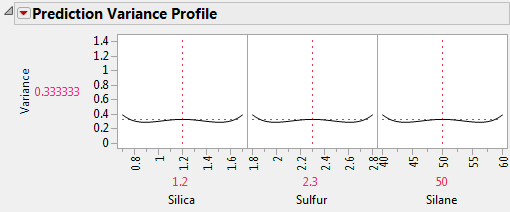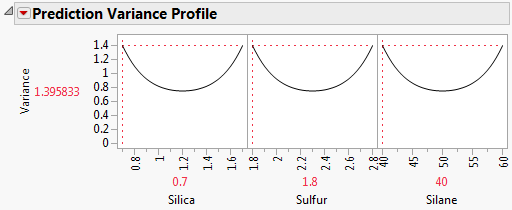Publication date: 11/10/2021

## Prediction Variance Profile

The Prediction Variance Profile helps you to understand where in the design space your predictions have more or less variability. Low prediction variance is desired. Use the Maximize Variance option to find the maximum variance. See Maximize Variance.

The Prediction Variance Profile plots the relative variance of prediction as a function of each factor at fixed values of the other factors. Figure 15.18 shows the Prediction Variance Profile for the Bounce Data.jmp data table, located in the Design Experiment folder.

Figure 15.18 Prediction Variance Profiler#### Relative Prediction Variance

For given settings of the factors, the prediction variance is the product of the error variance and a quantity that depends on the design and the factor settings. Before you run your experiment, the error variance is unknown, so the prediction variance is also unknown. However, the ratio of the prediction variance to the error variance is not a function of the error variance. This ratio, called the relative prediction variance, depends only on the design and the factor settings. Consequently, the relative variance of prediction can be calculated before acquiring the data. See Relative Prediction Variance.

After you run your experiment and fit a least squares model, you can estimate the error variance using the mean squared error (MSE) of the model fit. You can estimate the actual variance of prediction at any setting by multiplying the relative variance of prediction at that setting.

It is ideal for the prediction variance to be small throughout the design space. Generally, the error variance drops as the sample size increases. In comparing designs, you may want to place the prediction variance profilers for two designs side-by-side. A design with lower prediction variance on average is preferred.

#### Maximize Variance

You can evaluate a design or compare designs in terms of the maximum relative prediction variance. Select the Maximize Variance option from the Prediction Variance Profile red triangle menu. JMP uses a desirability function that maximizes the relative prediction variance. The value of the maximum variance in the Prediction Variance Profile is the worst (least desirable from a design point of view) value of the relative prediction variance. The maximum variance can occur at more than one combination of factor settings.

Figure 15.19 shows the Prediction Variance Profile with Maximize Variance selected. The plot is for the Bounce Data.jmp sample data table, located in the Design Experiment folder. The maximum value of the relative prediction variance is 1.3958 when Silica = 0.7, Sulfur = 1.8, and Silane = 40. However, keep in mind that several factor settings can have this same relative variance. The design point with Silica = 1.7, Sulfur = 2.8, and Silane = 60 also has relative prediction variance of 1.3958. To evaluate the prediction variance using a surface, see Prediction Variance Surface.

Figure 15.19 Prediction Variance Profile Showing Maximum Variance